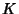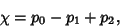## Euler Characteristic

Let a closed surface have Genus. Then the Polyhedral Formula becomes the Poincaré Formula(1)

whereis the Euler characteristic, sometimes also known as the Euler-Poincaré Characteristic. In terms of the Integral Curvature of the surface,(2)

The Euler characteristic is sometimes also called the Euler Number. It can also be expressed as(3)

whereis theth Betti Number of the space.